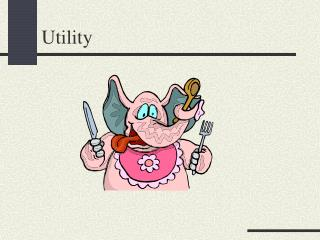DownloadDownload PresentationUtility

# Utility

Download Presentation## Utility

- - - - - - - - - - - - - - - - - - - - - - - - - - - E N D - - - - - - - - - - - - - - - - - - - - - - - - - - -
##### Presentation Transcript

1. Utility

2. The MRS is the slope of the indifference curve at a point MRS=derivative of indifference curve Marginal Rate of Substitution

3. Interpretation of MRS • The MRS measures the rate at which the consumer is willing (i.e., indifferent) to substitute one good for the other. • If good 2 is measured in dollars, the MRS measures the consumer’s willingness to pay for an extra unit of good 1.

4. Monotonicity: MRS negative (Strict) Convexity: MRS decreases as increases Assumptions on Preferences and the MRS

5. Utility Function • Idea: assign a number to each consumption bundle, with higher numbers assigned to more-preferred bundles. • A utility function represents a preference relation :

6. Utility Function: Does It Always Exist? • Q: Given a preference relation can we find a utility function that represents it? • A: If preferences are complete and transitive (plus a technical assumption called “continuity” is verified) we can. [Sufficient condition]

7. Is Transitivity Necessary? • Q: Is it necessary that preferences are transitive for the existence of a utility function that represents them? • A: Yes. Otherwise:

8. Utility is Just Ordinal E.g.: (1,1) (1,0.5) (0.4,1) (0.8,0.8) Can be represented in different ways:

9. In General If : • 1) represents • 2) is a positive monotonic transformation Then, also represents

10. Utility function: Indifference curves: or: Utility Functions and Indifference Curves

11. Utility function: Indifference curves: or: Indifference Curves and Monotonic Transformations

12. Utility function: Indifference curves: Perfect Substitutes

13. Utility function: Perfect Complements

14. Utility function: Indifference curves: Cobb-Douglas Preferences

15. Cobb-Douglas • Most commonly used utility function in economics • Possible to assume without loss of generality that • Why?: apply to get

16. Marginal Utility • Consider a consumer that is consuming • Q: By how much does his utility change as we increase by a very small amount his consumption of good 1? • A: Marginal utility of good 1:

17. Marginal Utility and Units • The marginal utilities of good 1 and good 2 depend on the specific utility function we are using. • Consider: • Then:

18. Marginal Utility and MRS • MRS only depends on preferences and not on their specific representation (utility function). • Marginal utilities can be used to compute the MRS between two goods.

19. Computing the MRS • Consider an indifference curve: • Q: What is the slope of this indifference curve (MRS)? • A:

20. The MRS and Monotonic Transformation • Consider a monotonic transformation • Q: What is the MRS in this case? • A: